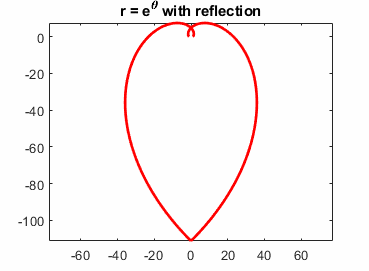MATLAB CommunityMATLAB, community & more

This is what happens when two logarithmic spirals love each other very much.

% Vary k from 1.5 to 3.5
k = 1.5;
t = linspace(0,k*pi,200);

r = exp(t);
x = r.*cos(t);
y = r.*sin(t);

plot(x,y,-x,y,'LineWidth',2,'Color',[1 0 0])
axis equal
set(gcf,'Color','white')
title('r = e^\theta with reflection')Happy Valentines Day!

|